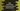# Dart: math library, its classes, constants and functions## dart:math library, its classes, constants and functions :

dart:math library is an inbuilt library in dart. It contains different mathematical constants, mathematical functions and a few classes. You can simply use this library by importing it in your code like below :

``import 'dart:math';``

In this post, I will show you these constants, functions and classes with a small description.

### Constants of dart:math :

Following constants are defined in dart:math :

#### 1. e(double) :

It is the base of natural logarithms. This is a double value. It is defined as :

``const double e = 2.718281828459045``

#### 2. ln2(double) :

This is the closest representable value of natural logarithm of 2. This is a double value and it is implemented as like below :

``const double ln2 = 0.6931471805599453``

#### 3. ln10(double) :

This is the closest representable value of natural logarithm of 10. It is also a double value.

``const double ln10 = 2.302585092994046``

#### log2e(double) :

Base 2 logarithm of e.

``const double log2e = 1.4426950408889634``

#### log10e(double) :

Base 10 logarithm of e.

``const double log10e = 0.4342944819032518``

#### pi(double) :

Constant π .

``const double pi = 3.1415926535897932``

#### sqrt1_2(double) :

Square root of half, 1/2 :

``const double sqrt1_2 = 0.7071067811865476``

#### sqrt2(double) :

Square root of 2.

``const double sqrt2 = 1.4142135623730951``

### Functions :

Following functions are defined in dart:math :

### Trigonometric functions :

#### 1. sin(num radians) → double :

Get the sine of the parameter radians. Result is NaN if radians is not finite.

#### 2. cos(num radians) → double :

Get the cosine of the parameter radians. Result is NaN if radians is not finite.

#### 3. tan(num radians) → double :

Get the tangent of the parameter radians. It is equal to sine(radians)/cos(radians). If radians is not finite, it will give NaN. If the value of cos is zero, it will give infinite result.

#### 4. asin(num x) → double :

Returns the arc sine of x in radians. If the value of x is not in between -1 to +1, it will return NaN.

#### 5. acos(num x) → double :

Get the arc cosine of x in radians.If the value of x is not in between -1 to +1, it will return NaN.

#### 6. atan(num x) → double :

Get the arc tangent of x in radians.It returns NaN, if the value of x is NaN.

#### 7. atan2(num a num b) → double :

It returns the angle in radians between the positive x axis and the vector (b,a). It returns the result in range -PI..PI. If any argument is NaN, it returns NaN.

### Other functions :

#### 1. sqrt(num x) → double :

Returns the positive square root of x. If the value of x is -0.0, it returns -0.0. For other negative values of x or NaN, it returns NaN.

#### 2. pow(num x num exponent) → num :

Returns x to the power of exponent.

#### 3. max(T a T b) → T :

Returns the larger between a and b. If any one of these is NaN, it returns NaN.

#### 4. min(T a T b) → T :

Returns the lesser between a and b. If any one of these is NaN, it returns NaN.

#### 5. exp(num x) → double :

Returns e to the power of x. If x is NaN, it returns NaN.

#### 6. log(num x) → double :

Returns the natural logarithm of x. If x is NaN or less than 0, it returns NaN. If x is equal to 0, it returns negative infinity.

### Classes :

Following classes are defined in dart:math library :

#### 1. MutableRectangle :

This class is for two dimensional axis aligned rectangles with mutable properties.

#### 2. Rectangle :

This class is to generate two dimensional rectangles with immutable properties.

#### 3. Random :

This class is to generate random values. It can be a random boolean, integer or double.

#### 4. Point :

This class is to generate a point in a two dimensional plan.